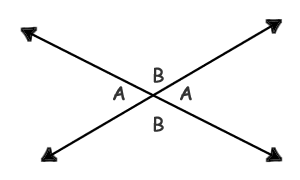# Vertical Angles

### Definition

Vertical angles are opposite from each other but share the same two lines and the same vertex.

### ExampleIn the above example, the B's share the same measure and the A's are the same as well. You can use this rule in more complicated problems where you need to find unknown angles. Look for any cases like this, where an angle is opposite from a known angle. Those are vertical angles, and will be equal.